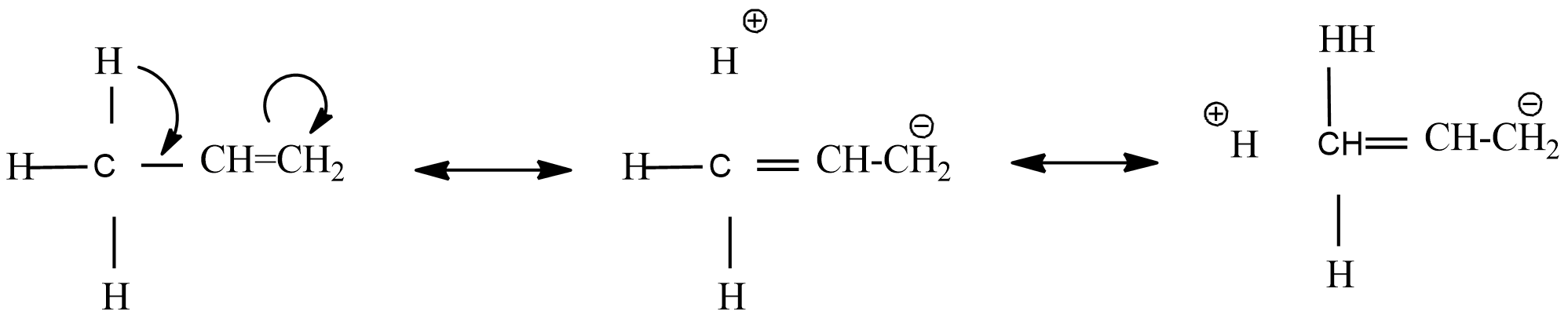QuestionAnswers

# Hyper conjugation is?(A) $\sigma -\pi$ Conjugation(B) Noticed due to delocalization of $\sigma -\pi$(C) no bond response(D) all the above

Hint: When an alkyl group is attached to an unsaturated system such as double bond or benzene ring, the order of inductive effect is actually reversed. This effect is called hyperconjugation effect, which involves delocalization of $\sigma$ -electrons through overlapping p-orbitals of double bond with $\sigma$-bond of the adjacent single bond.
Consider, the presence of $\alpha -H$ with respect to double bond, triple bond carbon containing positive charge in carbonium ion or unpaired electron with $\sigma -\pi$ conjugation.Hyper conjugation is the delocalization of $\sigma$ and $\pi$ bonds. From the above resonance structure, found that it ends in addition to bonds where there is no bond between C-H. Hence, there is no bond resonance.